Want to share your content on R-bloggers? click here if you have a blog, or here if you don't.

The Holt-Winters method is a popular and effective approach to forecasting seasonal time series. But different implementations will give different forecasts, depending on how the method is initialized and how the smoothing parameters are selected. In this post I will discuss various initialization methods.

Suppose the time series is denoted byand the seasonal period is(e.g.,for monthly data). Letbe the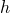-step forecast made using data to time. Then the additive formulation of Holt-Winters’ method is given by the following equationsell_t & = alpha(y_t – s_{t-m}) + (1-alpha)(ell_{t-1}+b{t-1})\
b_t & = beta(ell_t – ell_{t-1}) + (1-beta)b_{t-1}\
s_t &= gamma(y_t-ell_{t-1} – b_{t-1}) + (1-gamma)s_{t-m}\
hat{y}_{t+h|t} &= ell_t + b_th + s_{t+h-m},
end{align*}”title=”Rendered by QuickLaTeX.com” style=”vertical-align: 0px; border: none;”/>

and the multiplicative version is given byell_t & = alpha(y_t/ s_{t-m}) + (1-alpha)(ell_{t-1}+b{t-1})\
b_t & = beta(ell_t – ell_{t-1}) + (1-beta)b_{t-1}\
s_t &= gamma(y_t/(ell_{t-1} – b_{t-1})) + (1-gamma)s_{t-m}\
hat{y}_{t+h|t} &= (ell_t + b_th)s_{t+h-m}.
end{align*}”title=”Rendered by QuickLaTeX.com” style=”vertical-align: 0px; border: none;”/>

In many books, the seasonal equation (with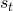on the LHS) is slightly different from these, but I prefer the version above because it makes it easier to write the system in state space form. In practice, the modified form makes very little difference to the forecasts.

In my 1998 textbook, the following initialization was proposed. Set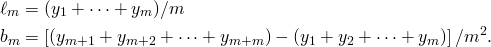ell_m & = (y_1+cdots+y_m)/m\
b_m &= left[(y_{m+1}+y_{m+2}+cdots+y_{m+m})-(y_1+y_2+cdots+y_{m})right]/m^2.
end{align*}”title=”Rendered by QuickLaTeX.com” style=”vertical-align: 0px; border: none;”/>

The level is obviously the average of the first year of data. The slope is set to be the average of the slopes for each period in the first two years: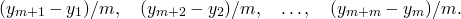(y_{m+1}-y_1)/m,quad (y_{m+2}-y_2)/m,quad dots,quad (y_{m+m}-y_m)/m.
]”title=”Rendered by QuickLaTeX.com” style=”vertical-align: 0px; border: none;”/>

Then, for additive seasonality setand for multiplicative seasonality set, where. This works pretty well, and is easy to implement, but for short and noisy series it can give occasional dodgy results. It also has the disadvantage of providing state estimates for period, so that the first forecast is for period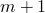rather than period 1.

In some books (e.g., Bowerman, O’Connell and Koehler, 2005), a regression-based procedure is used instead. They suggest fitting a regression with linear trend to the first few years of data (usually 3 or 4 years are used). Then the initial level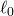is set to the intercept, and the initial slope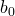is set to the regression slope. The initial seasonal values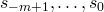are computed from the detrended data. This is a very poor method that should not be used as the trend will be biased by the seasonal pattern. Imagine a seasonal pattern, for example, where the last period of the year is always the largest value for the year. Then the trend will be biased upwards. Unfortunately, Bowerman, O’Connell and Koehler (2005) are not alone in recommending bad methods. I’ve seen similar, and worse, procedures recommended in other books.

While it would be possible to implement a reasonably good regression method, a much better procedure is based on a decomposition. This is what was recommended in my 2008 Springer book and is implemented in the `HoltWinters` and `ets` functions in R.

1. First fit amoving average smoother to the first 2 or 3 years of data (`HoltWinters` uses 2 years, `ets` uses 3 years). Here is a quick intro to moving average smoothing.
2. Then subtract (for additive HW) or divide (for multiplicative HW) the smooth trend from the original data to get de-trended data. The initial seasonal values are then obtained from the averaged de-trended data. For example, the initial seasonal value for January is the average of the de-trended Januaries.
3. Next subtract (for additive HW) or divide (for multiplicative HW) the seasonal values from the original data to get seasonally adjusted data.
4. Fit a linear trend to the seasonally adjusted data to get the initial level(the intercept) and the initial slope.

This is generally quite good and fast to implement and allows “forecasts” to be produced from period 1. (Of course, they are not really forecasts as the data to be forecast has been used in constructing them.) However, it does require 2 or 3 years of data. For very short time series, an alternative (implemented in the `ets` function in R) is to set the initial seasonal values to be the last year of available data with the mean subtracted (for additive HW) or divided out (for multiplicative HW). Then proceed with steps 3 and 4 as above.

Whichever method is used, these initial values should be seen as rough estimates only. They can be improved by optimizing them along with the smoothing parameters using maximum likelihood estimation, for example. The only implementation of the Holt-Winters’ method that does that, to my knowledge, is the `ets` function in R. In that function, the above procedure is used to find starting values for the estimation.

Some recent work (De Livera, Hyndman and Snyder, 2010) shows that all of the above may soon be redundant. In Section 3.1, we show that the initial state values can be concentrated out of the likelihood and estimated directly using a regression procedure. Although we present the idea in the context of complex seasonality, it is valid for any exponential smoothing model. I am planning on modifying the `ets` function to implement this idea, but it will probably have to a wait for a couple of months as my “to do” list is rather long.